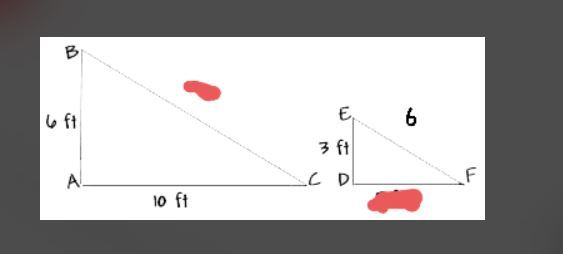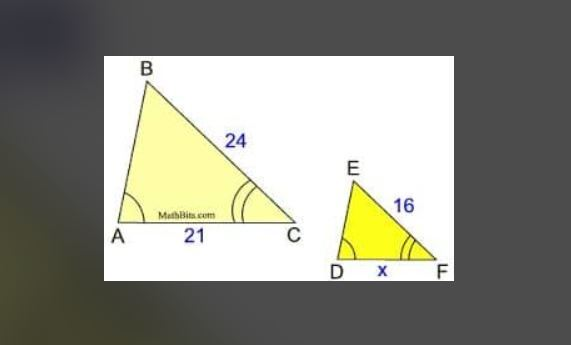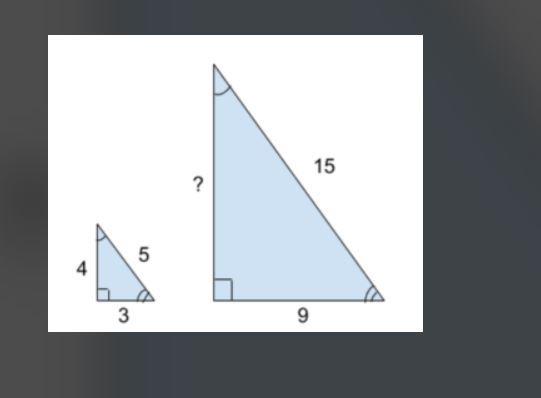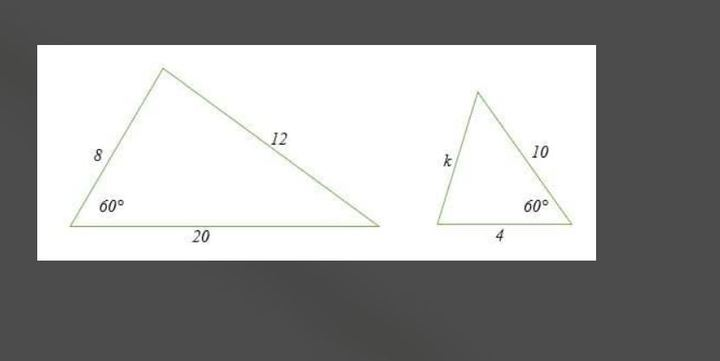## Forums » Mathematics

### Mathematics August, 2021 Journal

•• 24 posts
September 6, 2021 10:01 PM PDT

We took our first math free tutoring session on Aug 25th, 2021.  We had two students: one from 7th grade and the other 8th grade.

- He always memorizes his math and makes silly mistakes

- When homework is given, he copies older solutions and memorizes many things

- Lacks confidence in math, sometimes after working with a formula many times,  he might not be able to use that formula properly in a new problem.

- Guardians asked us to orally ask him question to evaluate him

- When new test was presented to him, he did not submit

"His teacher has given him homework from the previous chapters. But he was going to do it by looking at the old solutions if he stopped anywhere which i asked him not to do. So now he is going to do it all by himself and if he stops somewhere I asked him to ask his teacher the next day instead of seeing the solutions and trying to understand and then do it. Otherwise, there won’t be any way for evaluating if this student actually understood the chapter or not.

Can you please ask teacher to test him orally by going over a few of the maths from the homework in the first 5-10min of the class?"

"So from what i have noticed, he gets nervous to see something new. That be a new question with formulas he has worked with previously or a new formula itself. So all he needs is just to make him understand the problems in a very easy manner and make him practice that a lot. It would be great if his tutor can take a weekly or bi weekly exam with questions of his own. As he has a tendency of memorizing solutions."

"I think if the student could get his daily problems solved and teacher can ask him to solve similar questions related to the problems (not the ones in the book) student had faced , that would be helpful. Student lacks confidence in maths badly and tends to memorize questions by solving them over and over again. So it’s basically not specific chapters now but student can say more about it if there is something specific. A weekly test would be helpful too".

"I showed him a couple of maths today and I got a lot of silly mistakes. Such as, he will cross out 2 and 4 if the maths is like (4x+1)/2. And his answer will be 2x+1 which is just so silly".

Like if 1/2 is in front of (4x+1), he does that"

- She took a double promotion to 8th grade, but wanted to see 7th grade math.

- Similarly maths, esp how to find if shapes are congruent and algebra maths

- She can't remember the formulas of algebra and sometimes she has problems in solving equations too

Solution by the teacher:

- Gave some homework

- Prepared test and took test since that is where students needed to pace up and that was how it could be checked if they understood the concepts

Here are some screenshots of the test taken by the teacherThis post was edited by Fatima Ahmed at September 6, 2021 10:28 PM PDT